TitleBeginning Algebra
Answer/Discussion to Practice Problems
Tutorial 36: Practice Test on Tutorials 32 - 35

 Problems 1a - 1b: Solve each equation for the specified variable.

 1a.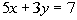for yAnswer: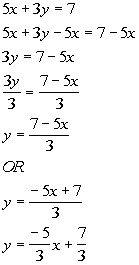1b.    I = PRT;   for PAnswer: Do you recognize this formula? This happens to be the formula for simple interest, where I = simple interest, P = principal, R = annual percentage rate, and T = time in years. In this problem we need to solve for P.  This means we need to get P on one side and EVERYTHING ELSE on the other side using inverse operations. Let's solve this formula for P: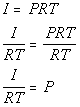Problems 2a - 2b: Solve the following word problems.

 2a.  A ramp 5 feet long is leaning against a raised platform which is 4 feet above the ground.  What is the distance from the ramp's contact point with the ground and the base of the platform?Answer: Since we are looking for the side of a right triangle,  we can use the Pythagorean formula: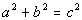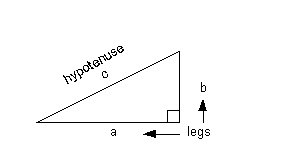The variables in this formula represent the following: a and b = legs of the right triangle c = hypotenuse of the right triangle   In this problem,  a = ? = this is the variable we are looking for b = 4 c = 5Plugging the values into the formula we get: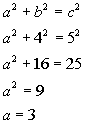FINAL ANSWER:  The distance from the ramp's contact point with the ground and the base of the platform is 3.

 2b.  A farmer has three cylindrical shaped containers to hold feed in.  Each container has the same radius of 3 feet and height of 4 feet.  What is the total volume of the three containers?Answer: Since we are needing to find the volume of a cylinder,  we can use this formula: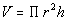The variables in this formula represent the following: V = volume of a cylinder r = radius h = height In this problem,  V = ? = this is the variable we are looking for r = 3  h = 4Plugging the values into the formula we get: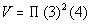First, find the volume of a cylinder: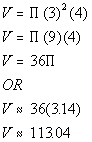Each cylinder has a volume of 36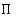cubic feet  or approximately 113.04 cubic feet.  If there are three of them, then we need to multiply this volume by 3.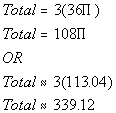The total volume for three cylinders is 108cubic feet  or approximately 339.12 cubic feet.

 Problem 3a: Answer the question on complimentary angles.

 3a.   What is the complementary angle to 47 degrees?Answer: Basically we need an angle that when we add it to 47 we get 90.  Let's set it up and solve it  algebraically, letting x be the missing angle and see what we get: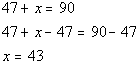The complimentary angle to 47 degrees is 43 degrees.

 Problem 4a: Answer the question on supplementary angles.

 4a.  What is the supplementary angle to 47 degrees?Answer: Basically we need an angle that when we add it to 47 we get 180.  Let's set it up and solve it  algebraically, letting x be the missing angle and see what we get: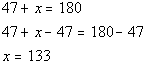The supplementary angle to 47 degrees is 133 degrees.

 Problem 5a: Answer the question on congruent figures.

 5a.  Figure ABCD is congruent to figure EFGH. If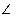B = 70,C = 55, andD = 25, what is the measure ofH?  If AD = 25, EF = 35, and BC = 12, what is the length of EH?Answer: SinceH corresponds withD and the figures are congruent, thenH =D = 25 degrees.   Since side EH corresponds to side AD and the figures are congruent, then side EH = side AD = 25.

 Problem 6a: Answer the question on similar figures.

 6a.   Figure ABCD is similar to figure EFGH. IfB = 60,C = 50, andD = 45, what is the measure ofG?  If AD = 25, EH = 50, and BC = 10, what is the length of FG?Answer: SinceG corresponds toC and the figures are similar, thenG =C = 50.   Since side FG corresponds to side BC and the figures are similar to each other, then FG and BC are in proportion to each other.  Similarly, EH and AD are in proportion to each other.  When setting up the proportion, make sure that you set it up the same on both sides.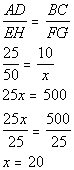FG = 20

 Problems 7a - 7d: Use the following figure to answer the questions.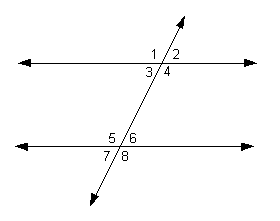7a.   Find2 if7 = 75 degrees.Answer: Since2 and7 are alternate exterior angles and the two lines are parallel, then2  =7 = 75 degrees.

 7b.  Find3 if6 = 75 degrees.Answer: Since3 and6 are alternate interior angles and the two lines are parallel, then3 =6 = 75 degrees.

 7c.  Find1 if5 = 105 degrees.Answer: Since1 and5 are corresponding angles and the two lines are parallel, then1 =5 = 105 degrees.

 7d.  Find4 if6 = 75 degrees.Answer: Since4 and6 are not alternate exterior, alternate interior or corresponding angles, they are not guaranteed to be equal.  However, since3 and4 make a straight angle (180 degrees) and3 and6 are alternate interior angles (which means they are equal), we can find the measure of4.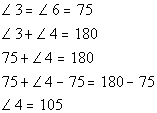4 = 105 degrees.

 Problem 8a: Find the mean, median, and mode.

8a.  The number of points a receiver has made during the last 5 games is given in the following table:

 Game game 1 game 2 game 3 game 4 game 5 Points 12 6 12 12 18

Find the mean, median, and mode of the points he scored in a game.Answer:

The mean is the average of the scores.

So we need to sum up all of the points and then divide by 5, since there are 5 games: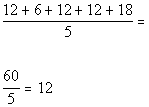The mean is 12.

The median is the middle value.

We need to list the numbers in numeric order:
6, 12, 12, 12, 18

Our median is going to be 12 (the second 6 listed).  That number has two values above it and two below it, so it is the middle value.

12 is the median.

The mode is the value that occurs the most often.

It helps to list the numbers in order to find the mode.
6, 12, 12, 12, 18

12 occurs three times, which is the most.

12 is the mode.

 Problem 9a: Find the test score.

 9a.  A student received scores of 62, 75, and 77 on three quizzes.  If tests count twice as much as quizzes, what is the lowest score that the student can get on the next test to achieve a mean of at least 70?Answer: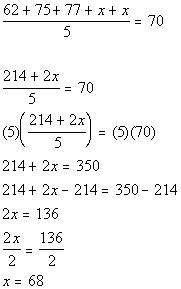The student would have to score a 68 on the next test to have a mean of 70.

 Problems 10a - 10b: Write the next three numbers in the sequence.

 10a.  1, 3, 7, 13, 21, ...Answer: My first inclination is to see if there is some pattern in addition.  Well, we are not adding the same number each time to get to the next number.   But, it looks like we have 1 +2, 3 +4, 7 +6, 13 +8, 21, .... I see a pattern in addition - do you see it?  We are always adding the next even number.  The pattern is to add the next even number.  The next three terms would have to be 31, 43, and 57, since 21+10 = 31,  31 +12 = 43, 43 +14 = 57.

 10b.  1, 5, 25, 125, ...Answer: When you see a big jump in numbers all of the sudden - we start small with 1, 5, and 25 then all of the sudden we are at 125 -  a good place to start is multiplication or exponents.  It is not a 100% rule, but it gives you a starting place.  It looks like we have 1 (5), 5  (5), 25  (5),  125,...There is a pattern in multiplication,  we are always multiplying by 5.    The pattern is to multiply the previous number by 5.  The next three terms would have to be 625, 3125, 15625, since 125(5) = 625,  625(5) = 3125, and 3125(5) = 15625.

 Problem 11a: Sara, Trudy, Jill and Karen all work for the same company.  One is a secretary, one is a manager, one is a computer programmer and one is a engineer.  Use the statements below to answer the question that follows.

 11a. A.   Sara and Jill eat lunch with the manager. B.   Trudy and Karen carpool with the secretary. C.   Sara likes to work out with the engineer and the secretary. Who is the secretary?Answer: You can use a process of elimination on this problem.  Statement A,  Sara and Jill eat lunch with the manager, doesn't let us definitively eliminate anyone from being the secretary. However, statement B,  Trudy and Karen carpool with the secretary, eliminates Trudy and Karen from being the secretary.  Statement C,  Sara likes to work out with the engineer and the secretary, eliminates Sara.  The only one that could be (100%, without a doubt) the secretary is Jill.

Last revised on August 2, 2011 by Kim Seward.
All contents copyright (C) 2001 - 2011, WTAMU and Kim Seward. All rights reserved.